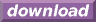#Calcute: freeware calculator

## Arccosine (arc-cosine, inverse cosine, acos)

The arc-cosine is the inverse of the cosine function. Calcute implements it with the acos function. The inverse cosine of a value x is the value y for which the cosine of y is x. In other words if y = acos(x) then x = cos(y). And since the cosine takes an angle as input parameter, the arccosine produces an angle as its output.

```acos(0.5)
1.0471975512
```

The acos value is expressed by Calcute using the currently-selected angle unit: radian, degree or gradient. In the above example, the result is in radians.

The arc-cosine function is only defined for parameter values in the close range [-1, +1] which corresponds to the output range of the cosine function. Note that the cosine function is periodic: its values repeat infinitely for angles that represent more than one complete rotation. Conversely, the arccosine is a multi-valued function: any parameter value in the valid range matches an infinite number of possible angles. But the acos function generates a single value refered to as the principal value. This is the "nearest" valid angle that remains within one revolution. All other possibilities can be obtained by adding or subtracting any whole number of complete rotations from the return value of acos.

In some mathematical texts and hand-held calculators, the notation cos-1 is sometimes used to represent the arc-cosine function. This is an unfortunate notational choice since it could also stand for the multiplicative inverse 1/cos, which is a different function.

Yet another term also used to represent the inverse cosine function is "arccos".